# C. Details of the Model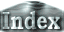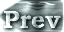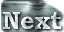### g. Vertical Filter

Vertical filtering is introduced in addition to the upper damping layer. The filter proposed by Shapiro (1972) is applied for u and v. However, if it is applied as is to the temperature, the smoothing procedure by the filter causes a global mean loss of approximately 150 W/m2 of energy

Therefore, the filtering described below is applied to temperature in the present study. First, a reference state is determined. Specifically, this state is determined using the values from a half-integer grid, Tk+1/2, as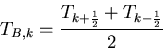For temperature, the values of Tk+1/2 are calculated within the model, thus are used directly. The smoothing procedure is performed so that Tk approaches the reference state. Adjustments are made starting at k = 2 as in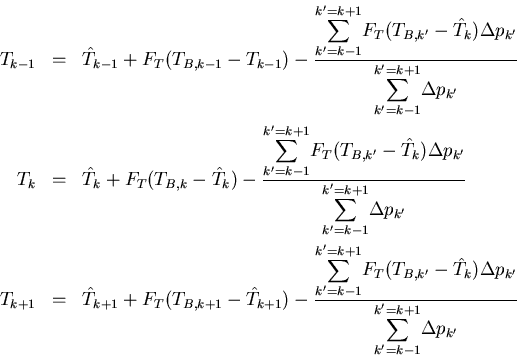and subsequently in order of increasing values of k up to k = 31. In the above equations,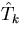represents the value of temperature prior to the filter-based adjustments.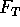is a coefficient that indicates the strength of the filtering. The purpose of the third terms in all the equations is to equally disperse, into three layers, the internal energy that is lost when temperature approaches the reference value at each layer height.

For the calculation, the value of the filtering strength coefficient for temperature,, is set to 0.1. In the case of S = 1500 W/m2, filtering is introduced for horizontal winds, and the value of the associated filtering coefficient is set to 0.2. In the actual 3D calculations, the amount of energy change caused by these filtering operations is small, and thus does not affect the energy budget.